## 3. 2. 5 Volume Integration

Hitherto, the boundary integrals have been discussed, while the integration of the termswas not mentioned at all. These integrals are usually on the associated dual cell of the vertex. In the following it is assumed that for the integration the functionalhas the form of a (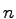-dimensional) Dirac pulse which is located in the geometrical point of the vertex.

Another method is to assume that the variable value is distributed uniformly on the cell. Even though such an interpretation of the quantity value is possible, it inevitably leads to an inconsistence: Given the Poisson equation, the functionalis the cell-wise constant right hand side of the equation and does not depend on the function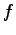. If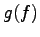is constant, the assumption of linear shape functions as chosen for the Laplace equation is inconsistent, because the Laplace operator applied to the shape function always yields zero.

Therefore, the integral is evaluated by multiplying the function value associated with the vertex with the volume of the dual cell of the vertex. If the functionaldepends on, e.g.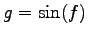, the evaluation of the cell is more complicated and not further discussed here. In most of the cases it is appropriate to approximate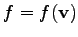throughout the dual cell of. Therefore, the following approximation can be derived: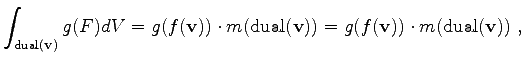(3.37)

where the function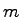denotes the volume of a given dual cell. For the sake of brevity, these two functions can be combined and one obtains the final formulation.

The distribution of quantities can be obtained from the topological view on the discretization scheme. All solution quantities which are required to form the solution function are associated with vertices. Furthermore, all quantities which are required to specify the functionare associated with the vertex.

Geometrical quantities are associated with the topological elements or their dual elements for which they are required. As an example, the volume of a cell (finite volume) is assigned to the vertex which is dual to the respective cell. The area of the dual surface of an edge is stored as an edge-based quantity. The length of an edge is also stored as edge-based quantity.

Michael 2008-01-16